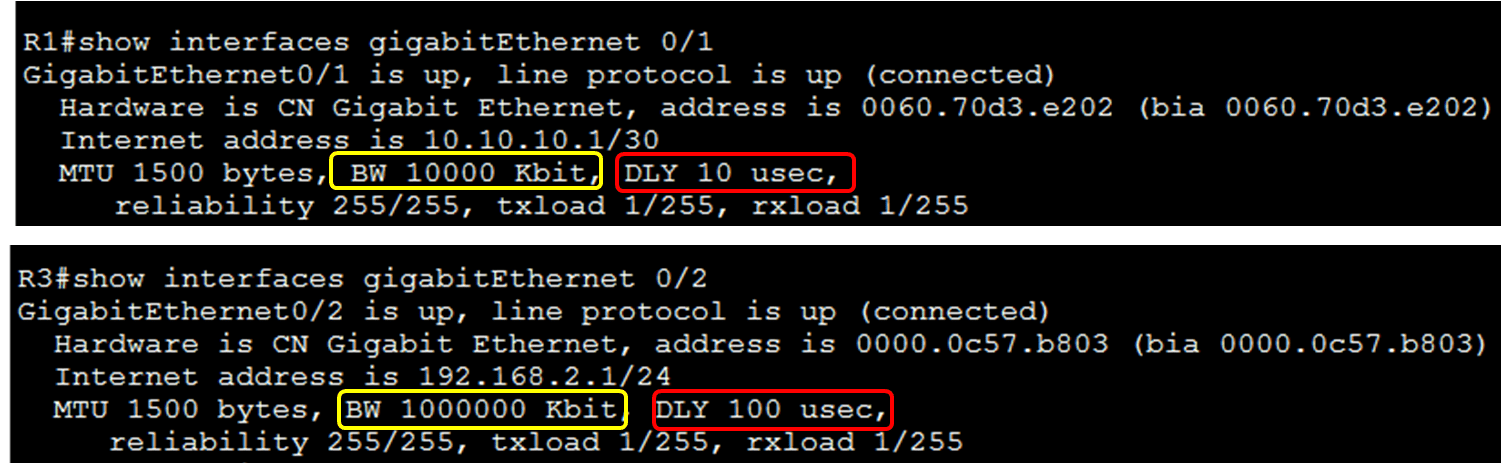# How to Calculate EIGRP Metric

EIGRP automatically calculates the routing table metric to choose the best path. But it is important that the network administrator understands how to calculate EIGRP metric. The default formula for the composite metric is:

• [k1 x Bandwidth + k3 x delay] x 256
• By default K1 and K3 is equal to 1 so the formula become
• [Bandwidth + Delay] x 256

After examining the bandwidth and delay values for all of the outgoing interfaces of the route, the steps to calculate EIGRP metric are the following:

1. Find out the link with the slowest bandwidth and use that value to calculate bandwidth using the formula 10,000,000/bandwidth.
2. Find out the delay value for all outgoing interface on the way to the destination. Sum up the delay values of all interfaces and divide by 10.
3. The sum of bandwidth and delay metric produce 24-bit value, but the EIGRP uses 32-bit value; therefore we multiply the 24-bit value with 256 to get 32-bit value.

## Calculating EIGRP Metric

Here in this example, we will use the same topology as used in earlier articles. This example displays how EIGRP determines the metric displayed in R1’s routing table for the 192.168.2.0/24 network.### Bandwidth

We have earlier discussed that EIGRP uses the slowest bandwidth to calculate the metric. We can find out the slowest bandwidth by examining each interface between the source and destination. So, in this example, the bandwidth of the interface between the source router R2 and the destination network 192.168.2.0/24 are illustrated in figure-2.The GigabitEthernet 0/1 interface on R1 has a bandwidth of 10000 kb/s. The GigabitEthernet 0/2 interfaces on R3 has a bandwidth of 1,000,000 kb/s. The bandwidth of the GigabitEthernet 0/1 interface on R1 is not the default bandwidth, because we have configured the bandwidth of this interface. So, the slowest bandwidth is 10000 kb/s and this bandwidth is used in the calculation of the metric.

The reference bandwidth of EIGRP is 10,000,000; the EIGRP divides this value by the interface bandwidth value in kb/s. The higher bandwidth gives lower metric value and the lower bandwidth produce higher metric value. So, 10,000,000 is divided by 10000 which results in 1000. If the result is not a whole number, then the value is rounded down.

### Delay

The same outgoing interfaces are used to find out the delay value, as shown in Figure 1. We know that the EIGRP uses the sum of all delays along the path from the source to the destination. The GigabitEthernet interface on R1 has a delay value of 10 usec. The GigabitEthernet 0/2 on R3 has a delay value of 100 usec. The sum of these delays is 110, which is divided by 10 (110/10 = 11).

## Calculate EIGRP Metric

Now we have calculated values for bandwidth and delay, so now we can calculate EIGRP metric. The simple formula for metric is [Bandwidth + Delay] x 256. To get the metric value we put the calculated values of bandwidth and delay in this formula for example [1000+11] x 256 = 258816. Now we can verify the calculated metric in the routing table for network 192.168.2.0/24. Figure 3 illustrates the output of the “show ip route” command.(Visited 97 times, 97 visits today)